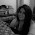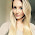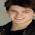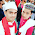## Friday, December 23, 2016

### Hello world.

I'm a low-dimensional topologist trying to learn the basics of machine learning + AI, especially the mathematics behind neural networks.

(Aside: This is just in case the title of the blog rings a too-distant bell.)

If you'd rather just tune me out and explore on your own, what little I do know I learned from the following sources, listed roughly in chronological order. As I learn more, I'll expand this list:
• Andrew Ng's Coursera course (also a really great place to get a broad overview of machine learning in general - beware: calculus is not a prereq, so the math is mostly black-boxed, but someone comfortable with multivariable calculus and linear algebra can fill most of those boxes with little trouble)
• Michael Nielsen's awesome free on-line Neural Networks and Deep Learning book
• Chris Olah's blog, colah's blog
• Jesse Johnson and his blog, The Shape of Data
• Mark Hughes

I'll begin with some basics about machine learning algorithms in general and neural networks in particular. I'm really going to start from the very beginning (a very good place to start, according to Julie Andrews). I feel a little funny doing this, because I'll start by saying some things which are probably pretty obvious to most people that have thought about this at all. OTOH, sometimes it helps to hear the obvious stuff. And there's a reasonable chance that many people who actually use neural nets have never really thought about the obvious stuff. Why take a chance?

I'll start by describing a particular class of problems machine learning algorithms like to try to solve.

Decision problems

According to wikipedia, a decision problem is a problem in some formal system with a yes or no answer, depending on the values of some input parameters. A canonical example is the problem of spam detection. Given an e-mail message, you'd like to classify it as "Yes - spam" or "No - not spam."

Of course, when we put it like this it is not yet a formal decision problem, because we haven't yet chosen a model. I.e., we haven't translated our floppy real-world question into a precise mathematical one, since we haven't determined what input parameters we will use to make the decision.

(Indeed, this is by far the hardest step. But let's roll with it anyway.)

So, step one is choosing some appropriate input parameters. Off the top of my head these might include, e.g.:
1. length (in characters) of the message,
2. number of misspelled words,
3. number of appearances of the word "sex",
4. what-have-you
At the moment, I think it's mostly humans that are charged with picking appropriate input parameters, but I do think humans are working on outsourcing this part to machines, too. Not sure yet how or how successfully.

Once the input parameters are chosen, you have formally defined your decision problem. You have yourself a multi-dimensional space, each axis of which corresponds to one of the input parameters you've chosen. Now each e-mail message can be assigned a point in this multi-dimensional space, and you're in business.

All you need now is to take the data you have (which, perhaps, a human has gone through and manually classified as "Yes - spam" or "No - not spam") and develop from it an algorithm to partition your parameter space into a "Yes" part and a "No" part. The idea here is that if you now get a new e-mail message that you haven't seen and assign it a point in your parameter space, your algorithm will now classify it as "Yes" or "No" depending on where it lands.

So what do you want your algorithm to do? Well, it's reasonable to guess that if some point is classified as "Yes" then nearby points will be too. So your job is to figure out how to build some (one? two? not sure) walls in your parameter space to separate the "Yes" sections from the "No" ones. These walls are often called the decision boundaries.

What's the easiest way we can possibly imagine our space being partitioned? (Besides everything being classified as "No", obviously...) That's right! Split in two by a hyperplane. This is the linearly separable situation, and it's a particularly happy one, so we'll talk about it first.

When your decision boundary is a single hyperplane (your data is linearly separable)

Recall that a hyperplane is just a multi-dimensional analogue of a plane imbedded in 3-dimensional space or a line imbedded in 2-dimensional space. In general, if our parameter space is n-dimensional, a hyperplane is an (n-1)-dimensional linear space that cuts your n-dimensional space neatly in two.

And the nice thing about a hyperplane in n-dimensional space is that it is the solution set of a single linear equation in n variables. In linear algebra terms, it is the set of vectors $\vec{x} = (x_1, \ldots, x_n) \in \mathbb{R}^n$ whose dot product with a particular vector $\vec{a} = (a_1, \ldots, a_n) \in \mathbb{R}^n$ is a particular fixed value, $b$. That is, the hyperplane determined by a vector $\vec{a}$ and a shift $b$ is the set of vectors $\vec{x} \in \mathbb{R}^n$ for which $\vec{a}\cdot \vec{x} = b.$

The right way to understand this is to notice that a hyperplane through the origin of $\mathbb{R}^n$ is defined as the set of points whose dot product with a particular vector $\vec{a} \in \mathbb{R}^n$ is 0. That is, it is the (n-1)-dimensional subspace of vectors perpendicular or orthogonal to $\vec{a}$. Also note that $\mathbb{R}^n$ is completely filled up by the translates or shifts of this hyperplane through the origin, and since the dot product of any vector $\vec{x} \in \mathbb{R}^n$ with $\vec{a}$ is simply the signed length of its projection in the direction of $\vec{a}$ (in the case $\vec{a}$ has length $1$--in general, it is the signed length of this projection scaled by the length of $\vec{a}$), the hyperplane which is shifted by $b$ from the origin is precisely the set of vectors whose dot product with $\vec{a} \in \mathbb{R}^n$ is $b$.

Even better, if you believe that your decision boundary is a hyperplane determined by orthogonal vector $\vec{a}$ and shift $b$, then the algorithm for solving the decision problem is suuuuuuper simple: If $\vec{a}\cdot \vec{x} > b$, then "Yes." If $\vec{a} \cdot \vec{x} < b$, then "No."

Great!

Extracting confidence ratings from linearly separable data

Of course, in the linearly separable situation, there's always the possibility that you'll have a data point land right on the decision boundary hyperplane. Also, data is noisy and so it's reasonable to have lower confidence about your classification of data points that are very close to the decision boundary. This is where sigmoid functions, like $\frac{1}{1+ e^{-t}}$ are useful. A sigmoid function maps $0$ to $0.5$, negative values to the interval $(0,0.5)$, positive values to the interval $(0.5,1)$. Moreover, a sigmoid function converges quickly to $0$ for negative $t$ and quickly to $1$ for positive $t$.

So in practice what a sigmoid function does is translate the position of a point with respect to the decision boundary to a number that can be interpreted as the probability that the answer is "Yes." If you're right on the decision boundary, that number is 0.5 (50% certainty that they answer is "Yes"-makes sense!), and as you get further and further away from the decision boundary, your confidence in your answer increases. On one side, the probability approaches $1$ quickly (i.e., you quickly gain confidence that the answer is "Yes"), and on the other side, the probability approaches $0$ quickly (i.e., you quickly gain confidence that the answer is "No").

Upshot: Let $\sigma(t) := \frac{1}{1 + e^{-t}}$. If your data is linearly separable by a hyperplane defined by vector $\vec{a} \in \mathbb{R}^n$ and shift $b \in \mathbb{R}$, and you want to determine the "probability" that a particular point $\vec{x} \in \mathbb{R}^n$ corresponds to a "Yes" answer, just calculate $\sigma(\vec{a}\cdot\vec{x} - b).$

Note: the "noisier" you believe your data is, the farther away you will need to move from the decision boundary before you are truly confident in your assessment. In other words, the shallower you will want to make the "S" in the graph of your sigmoid function. Do this by replacing $-t$ with $-ct$ for $0< c<1$. Likewise, if you believe your data is less noisy, choose $1<c$ to make a your sigmoid function more closely resemble a step function.

More importantly, the assumption that your data is linearly separable is really much too strong in most cases. In most situations, assuming this will lead to pretty poor results. I mean, who's to say that the "Yes" part of your space is even connected? Or contractible? Maybe it has interesting topology. Who the heck knows? But it's a reasonable first pass for a newbie.

OK, that's probably enough for a very first post. Next time, I'll actually explain what a machine learning algorithm does in the linearly separable situation, in preparation for talking a bit about neural networks and trying to get at what they're up to.

1.Eli, I love this! I have not done math in a really long time, but you've explained it all so well. Glad you're writing this :)

1.Machine Learning Projects for Final Year machine learning projects for final year

Deep Learning Projects assist final year students with improving your applied Deep Learning skills rapidly while allowing you to investigate an intriguing point. Furthermore, you can include Deep Learning projects for final year into your portfolio, making it simpler to get a vocation, discover cool profession openings, and Deep Learning Projects for Final Year even arrange a more significant compensation.

Python Training in Chennai Python Training in Chennai Angular Training Project Centers in Chennai

2.In general, $c$ doesn't have to be less than 1, right? You could replace $-t$ with $-ct$ for any $c > 0$, with $c$ representing the distance from the separating hyperplane that represents about 73% confidence.

3.(Make that the reciprocal of the distance, so that $ct = 1$ at that distance.)

4.This comment has been removed by the author.

5.You're right that in general c does not have to be less than 1, as long as c>0. But if we want the S in the sigmoid function to be shallower than the standard sigmoid function (approach 1 more slowly as t approaches infinity and 0 more slowly as t goes to negative infinity, we want 0<c<1. If we want it to be steeper than the standard function, we select 1<c.

6.This company will always be there to withdraw your web money, perfect money and bitcoin fund through bitcoin atm card . This company not only facilitates you with its services but also understands the stress that you go through while exchanging your e-money.

7.8.Thanks for sharing your knowledge related computer, if anybody having issues in QuickBooks Accounting software call at QuickBooks Helpline Number Canada +1-209-337-3009 https://bit.ly/2GM9AVQ

9.Nice post.Thanks for sharing this post. Machine Learning is steadily moving away from abstractions and engaging more in business problem solving with support from AI and Deep Learning. With Big Data making its way back to mainstream business activities, to know more information visit: Pridesys IT Ltd

10.This comment has been removed by the author.

11.Full control over your funds while perform transactions with bitcoin exchanger trusted fast website working as highest degree of security privacy and professionalism

12.13.14.15.16.17.18.Good Post. I like your blog. Thanks for Sharing
Machine Learning Course in Noida

19.computer places near me

Welcome to WillPowerPCS. Get online used and new computers for sell. WillPowerPcs is a best places for quality computer repairs. Contact us - 518-892-4419

https://willpowerpcs.com/

20.21.Hi, I just gone through this blog, the information was really very valuable, would love to see more from you. i have also plenty of the same kind of content You can explore my blog by clicking the link below
Best digital marketing institute in delhi
Best digital marketing course in delhi
Digital marketing institute in delhi
Digital marketing course in delhi

call us: 9212265265

22.Top ranking seo specialist highly experienced with proven success locally registered company in canada europe usa for search engine marketing organic package

23.Microsoft Office Suite of items created by Microsoft that incorporates Microsoft Word, Excel, Access, PowerPoint, and Outlook. Get some simple strides for simple downloading, introducing, enacting, and re-introducing the Microsoft Office suite by visiting the accompanying connections.
office.com/setup

24.25.26.